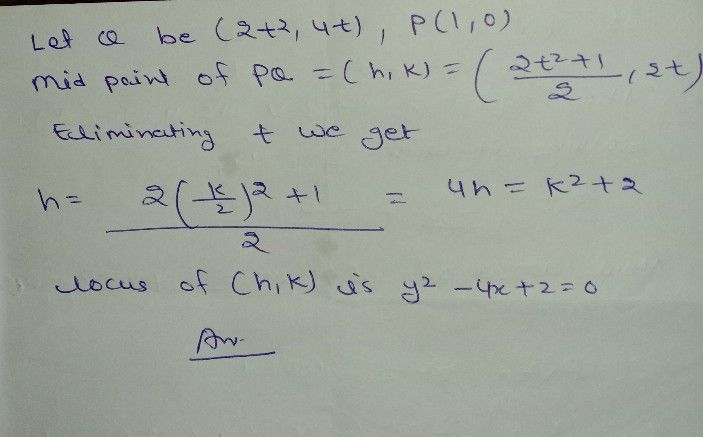Symbol
Problem$411$ $7$ be the point $11$ $0\right)$ and $C$ Q a point on the locus $x^{2}=8x$ The locus of the mid point of PQ is $13\right)$ $2+4y+2=0$ (b) $x^{2}-4x+2=0$ $0$ $x^{2}-4x+2=0$ $d\right)$ $2+4x+2=0$
Algebra
SolutionQanda teacher - AnushkaStudent
tnx